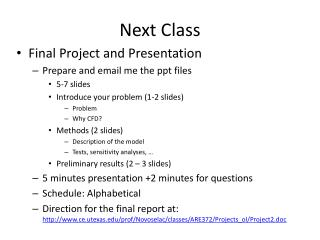DownloadDownload PresentationNext Class

# Next Class

Download Presentation## Next Class

- - - - - - - - - - - - - - - - - - - - - - - - - - - E N D - - - - - - - - - - - - - - - - - - - - - - - - - - -
##### Presentation Transcript

1. Next Class • Final Project and Presentation • Prepare and email me the ppt files • 5-7 slides • Introduce your problem (1-2 slides) • Problem • Why CFD? • Methods (2 slides) • Description of the model • Tests, sensitivity analyses, … • Preliminary results (2 – 3 slides) • 5 minutes presentation +2 minutes for questions • Schedule: Alphabetical • Direction for the final report at: http://www.ce.utexas.edu/prof/Novoselac/classes/ARE372/Projects_ol/Project2.doc

2. Lecture Objectives • Learn about Star-CCM+ and ANSYS-Fluent • Presenter Shichao Liu • Meshing • UDF • …. • Talk about parallel computing • Review course topics

3. Commercial: Fluent (Airpak) www.fluent.com/ Star CD www.cd-adapco.com/ Phoenics www.cham.co.uk/ Ansys CFX http://www-waterloo.ansys.com/ Flomerics (Flowvent) www.flomerics.com/ and many other Some free research: NIST Smokeview www.fire.nist.gov/fds/download/ ASHRAE - zero equation (published only source code) CFD software

4. Parallel methods • Big linear systems your project full-blown 3D problem! • More than 1 million nodes. • Memory becomes a problem – cannot store a matrix on one processor. • Slow calculation! • solution : use many processors! Split matrix to 4 processors in this example! p1 p2 p3 p4 BIG matrix

5. Course Masterial Review • Navier-Stokes equations and RANS • Turbulence modeling • Discretization and equation solver • Boundary conditions • Numerical properties • Specific CFD problems

6. Conservation Equations Navier Stokes Equations

7. Reynolds Averaged Navier Stokes equations Continuity: 1) Momentum: 2) 3) 4) Similar is for STy and STx 4 equations 5 unknowns → We need to model

8. Modeling of Turbulent Viscosity Fluid property – often called laminar viscosity Flow property – turbulent viscosity MVM: Mean velocity models TKEM: Turbulent kinetic energy equation models Additional models: LES: Large Eddy simulation models RSM: Reynolds stress models

9. Discretization and equation solver SIMPLE algorithm Discretization of RANS Guess p* p=p* Step1: solve V* from momentum equations Step2: introduce correction P’ and express V = V* + f(P’) Step3: substitute V into continuity equation solve P’ and then V Step4: Solve T , k , e equations no Converged (residual check) yes end

10. Summary of Course Objectives • Recognize the physics behind various numerical tools used for solving airflow problems. • Employ basic numerical methods for solving Navier-Stokes Equations. • Apply CFD for airflow simulations in buildings and use these tools in design and research. • Evaluate the thermal comfort and indoor air quality (IAQ) with different ventilation systems. • Assess human exposure to different pollutant types. • Critically analyze and evaluate CFD results.